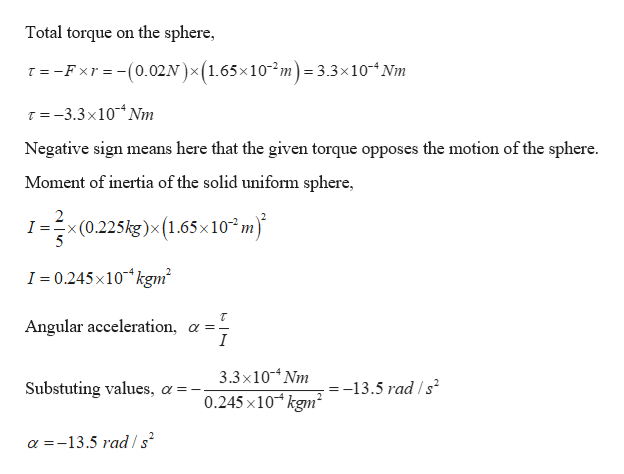# A machine part has the shape of a solid uniform sphere of mass 225 g and diameter 3.30 cm . It is spinning about a frictionless axle through its center, but at one point on its equator it is scraping against metal, resulting in a friction force of 0.0200 N at that point.Find its angular acceleration. Let the direction the sphere is spinning be the positive sense of rotation.Express your answer in radians per second squared.

Question
45 views

A machine part has the shape of a solid uniform sphere of mass 225 g and diameter 3.30 cm . It is spinning about a frictionless axle through its center, but at one point on its equator it is scraping against metal, resulting in a friction force of 0.0200 N at that point.

Find its angular acceleration. Let the direction the sphere is spinning be the positive sense of rotation.
check_circle

Step 1

Given:

Mass of the sphere = 225 g = 0.225 kg

Diameter = 3.30 cm  or Radius = 1.65 cm

Force in equator = 0.02 N

Step 2

Calcualting the angular accel...help_outlineImage Transcriptioncloseon the sphere Total torque T =-Fxr -(0.02N)x (1.65x 10-2m) = 3.3x10Nm T =-3.3x10 Nm Negative sign means here that the given torque opposes the motion of the sphere Moment of inertia of the solid uniform sphere, 2 I: x(0.225kg)x(1.65x 10 m) I 0.245x10 kgm2 Angular acceleration, a =- 3.3x10 Nm 0.245 x10 kgm2 Substuting values =-13.5 rad /s2 =_ X a-13.5 rad /s fullscreen

### Want to see the full answer?

See Solution

#### Want to see this answer and more?

Solutions are written by subject experts who are available 24/7. Questions are typically answered within 1 hour.*

See Solution
*Response times may vary by subject and question.
Tagged in

### Rotational Mechanics